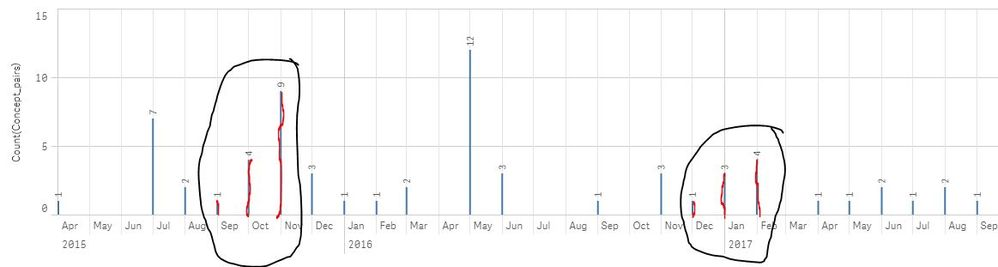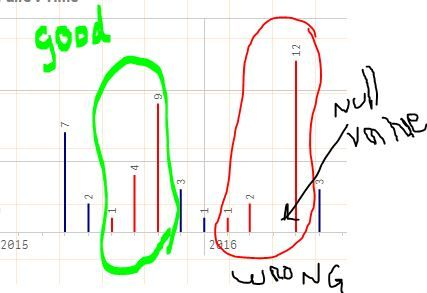# Qlik Sense App Development

Announcements
Uploads getting stuck in the virus scanner. We are investigating.
cancel
Showing results for
Did you mean:
HighlightedContributor II

## How to change histogram bar color dynamically with expression

Hi,

I've been trying to write an expression that changes the histogram bar to the color red whenever there is 3 months of consistent growth.  I'm new at Qlik Sense so please have patience with me.

Is it possible that this can happen dynamically when the histogram changes when the data filters are changed?

Below in the capture of what I'm trying to achieve.  Any help would be greatly appreciated.

Thanks

RichLabels (3)

• ### Qlik Sense

3 Replies
HighlightedMVP

Hi Rich, maybe using a straighforward query using Above and Below based on expression.
In attachement check color expression to see an example.

Well it doesn't allow me to upload qvf

Script was:

dim, value
1,5
2,7
3,1
4,3
5,5
6,7
7,5
8,1
9,5
10,7
11,3
];

Expression:

Sum(value)

Expression Label: Value

And color expression:

If(([Value]>Above([Value]) and [Value]>Above([Value], 2))
or ([Value]>Above([Value]) and [Value]<Below([Value]))
or ([Value]<Below([Value]) and [Value]<Below([Value], 2))
, LightRed(), blue())

HighlightedContributor II

I tried your solution and it worked well, but the only thing I noticed was it doesn't account for months with null values.  I understand that it looks at the rows above and below and if there is no input for that month then it doesn't show up in the table.

Is there a way to account for this?HighlightedMVP

Hello, creating a master calendar can help on having all months in x axis, this way null value can turn into 0 if expression is a sum().

Also the expression neded some adjustments:
If(([Value]>Above([Value]) and [Value]>Above([Value], 2) and Above([Value])>Above([Value], 2))
or ([Value]>Above([Value]) and [Value]<Below([Value]))
or ([Value]<Below([Value]) and [Value]<Below([Value], 2) and Below([Value])<Below([Value], 2))
, LightRed(), blue())